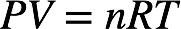# Problem: An 11.2-L sample of gas is determined to contain 0.50 mole of N2. At the same temperature and pressure, how many moles of gas would there be in a 20.-L sample?

###### FREE Expert Solution

Recall: The ideal gas law is as follows:The volume and moles of a gas are related to the pressure, temperature, and the universal gas constant:The value RT/P is constant. For a given gas, the initial and final volume and number of moles of the gas are related by Avogadro's law.94% (54 ratings)###### Problem Details

An 11.2-L sample of gas is determined to contain 0.50 mole of N2. At the same temperature and pressure, how many moles of gas would there be in a 20.-L sample?